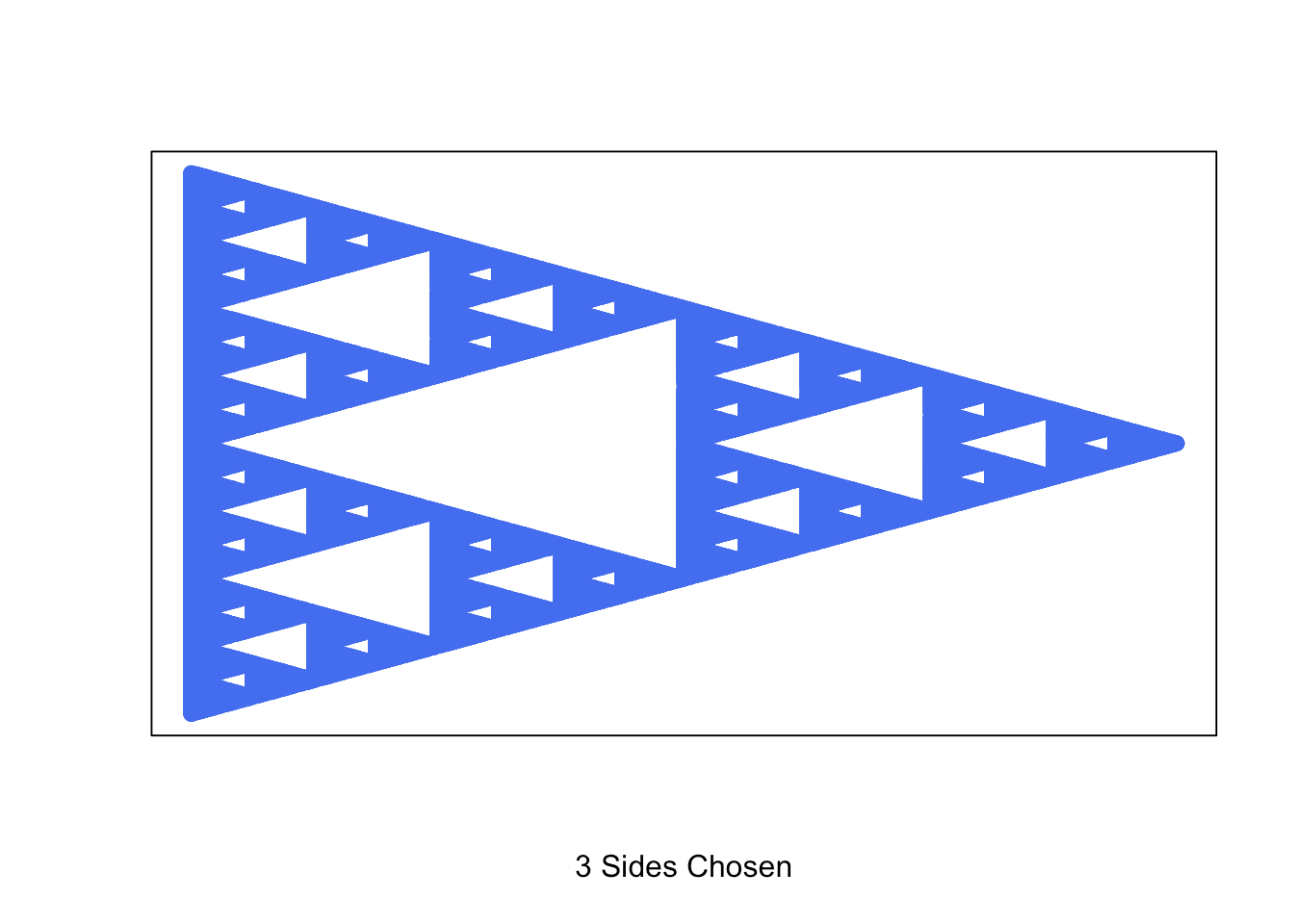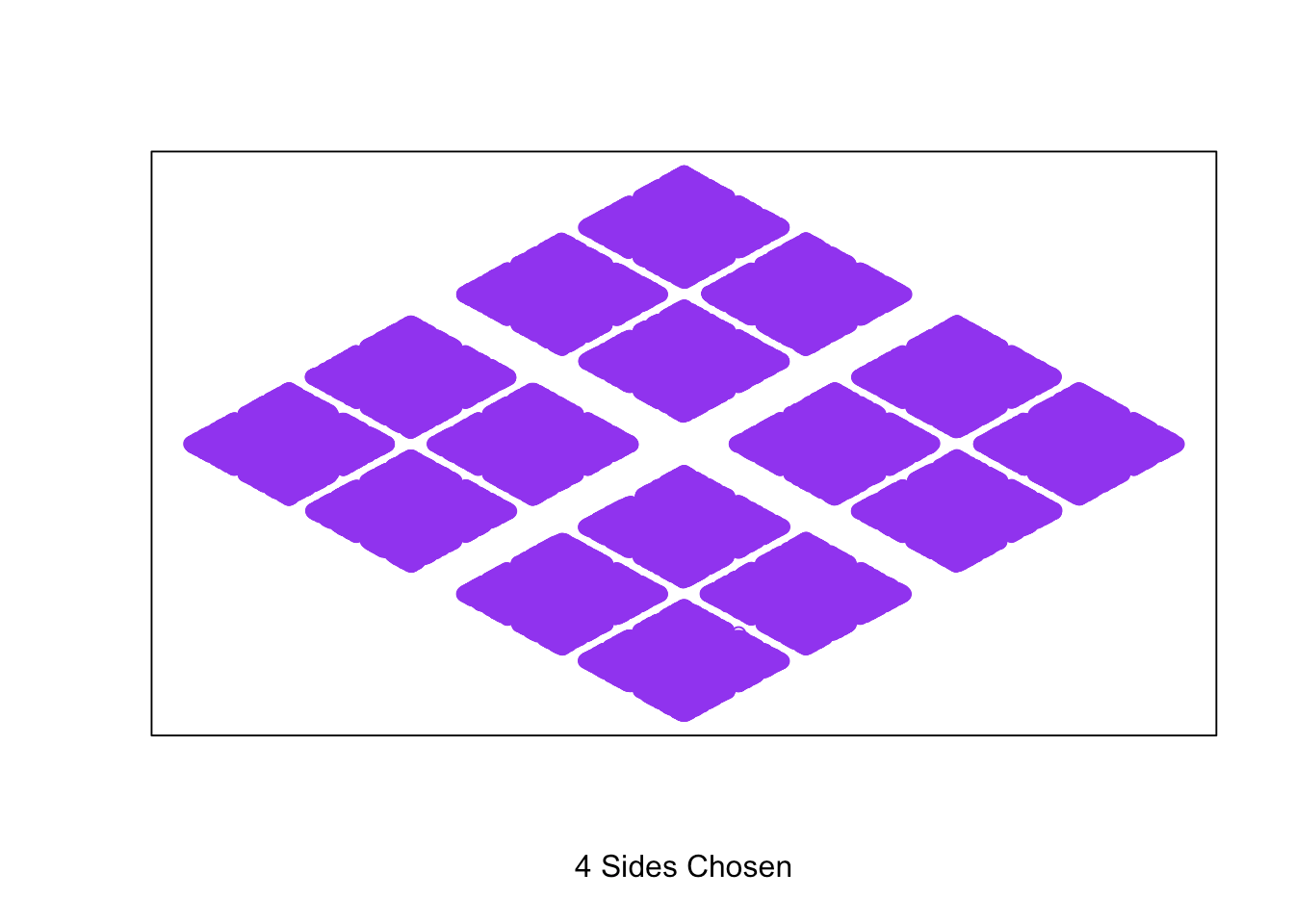Want to share your content on R-bloggers? click here if you have a blog, or here if you don't.

### Intro

This function allows us to generate the Sierpinski Triangle and explore other recursive shapes with equal length sides following the same algorithm.

```shape <- function(corners, trials = 100000){

corners <- as.integer(corners)
points <- list()

if (corners < 3) stop("Value should be 3 or greater")

for (n in 1:(corners)){
points\$x[n] <- 0 + cos((2*pi*n)/corners)
points\$y[n] <- 0 + sin((2*pi*n)/corners)
}

x <- points\$x
y <- points\$y

trials <- trials
sierpinski <- list()

for (t in 1:trials){
r <- sample(1:corners,1)
x <- (x + points\$x[r]) / sqrt(corners + 1)
y <- (y + points\$y[r]) / sqrt(corners + 1)
sierpinski\$x[t] <- x
sierpinski\$y[t] <- y
}

# I use these colors for random color selection. Update for your own desired selection.
color <- sample(c("royalblue2", "firebrick2", "gold2", "springgreen3", "purple2", "darkorange1"),1)

plot(sierpinski\$x[corners:trials], sierpinski\$y[corners:trials],
xlab = paste0(corners, " Sides Chosen"), ylab = "", xaxt = "n", yaxt = "n", col = color)

}```

When you run the function, you indicate the number of sides for the polygon and adjust the number of trials to change the resolution if desired. Here are some examples:

`shape(3)``shape(4)``shape(6)`